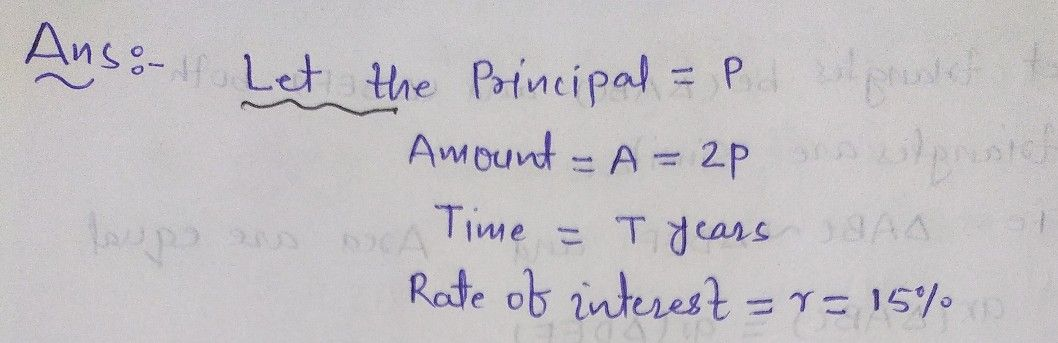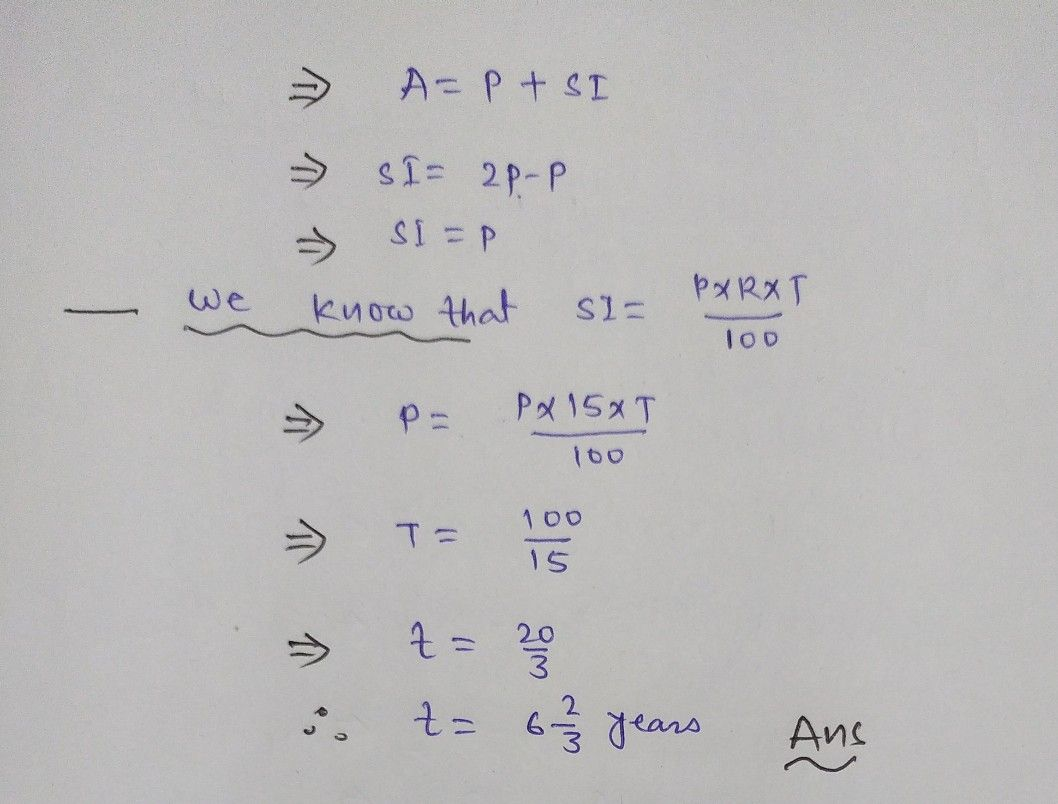Symbol
Problem$①$ money $\left(n$ what time will $a$ double itself $af$ sum $\bar{\infty }$ $5$ $0^{+}$